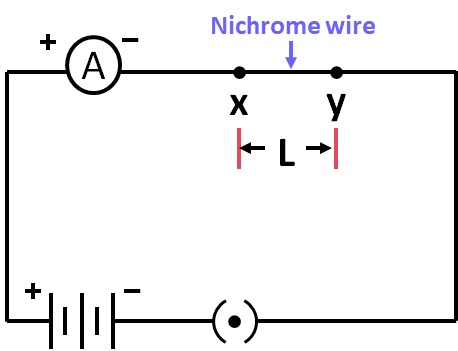Case Based Questions (MCQ)

Class 10
Chapter 12 Class 10 - Electricity

## In the given circuit, connect a nichrome wire of length ‘L’ between points X and Y and note the ammeter reading Answer any four questions from (a) to (e).## (iv) No change in ammeter reading

Resistance is directly proportional to length of the conductor.

So, if length is increased , resistance increases .

If resistance increases, current decreases .

Therefore, the ammeter reading will decrease.

So, the correct answer is (ii).

## (iv) No change in ammeter reading

Resistance of a conductor is inversely proportional to area of cross section.

So, if the area of the cross section increases , resistance decreases.

If resistance decreases, current increases .

Therefore, the ammeter reading will increase.

So, the correct answer is (i).

## (iv) 1:1

In a series connection of resistors, the same current passes through all the resistors.

Hence, the current will be the same.

So, Ratio of the currents will be 1: 1

So, the correct answer is (iv).

## (iv) manometer

An instrument that measures electric current in a circuit is an Ammeter.

So, the correct answer is (i).

## (iv) None of the wire will get heated up.

Heat produced, H = I 2 R

Where,

• I is the current
• R is the resistance

In a series circuit, the same current passes through both the wires.

So, Heat produced is directly proportional to the resistance.

For the same length and same radius, the resistance of nichrome wire is more than copper wire due to its higher resistivity.

Hence, Nichrome wire is heated up more than copper wire.

So, the correct answer is (i).

Learn in your speed, with individual attention - Teachoo Maths 1-on-1 Class